# 比特币白皮书中文版（刘教链译本）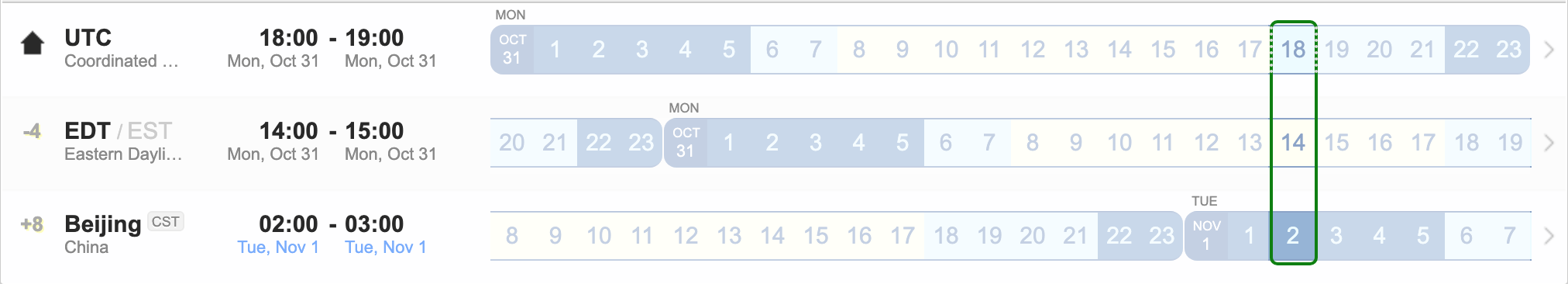2008年美东时间10月31号下午2点10分（北京时间11月1号凌晨2点10分），一个自称叫做Satoshi Nakamoto（中本聪）的账号向mezdowd的密码学邮件组投递了一封电子邮件，邮件标题叫做：Bitcoin P2P e-cash paper（比特币点到点电子现金论文）。在邮件正文中，他附上了这篇论文的pdf版本下载链接，并简述了论文的中心思想。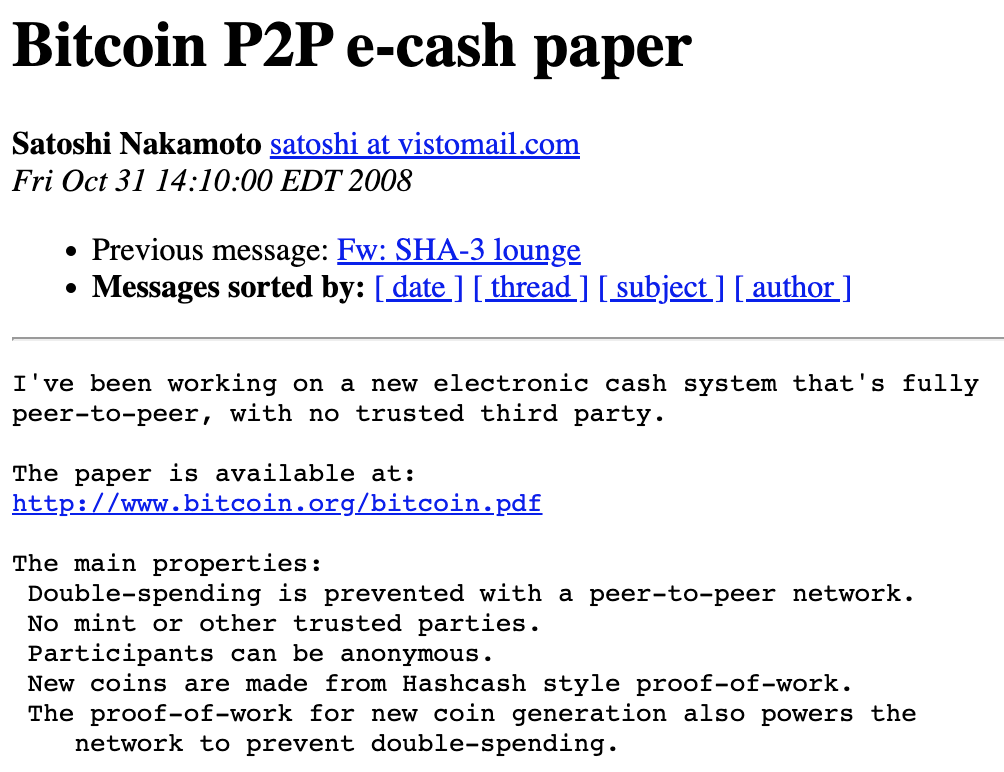1. 引言

2. 交易3. 时间戳服务器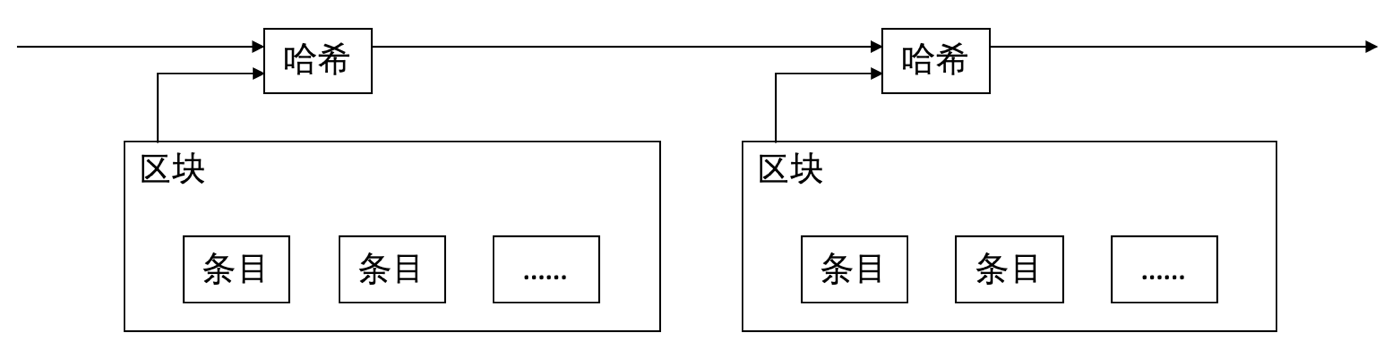4. 工作量证明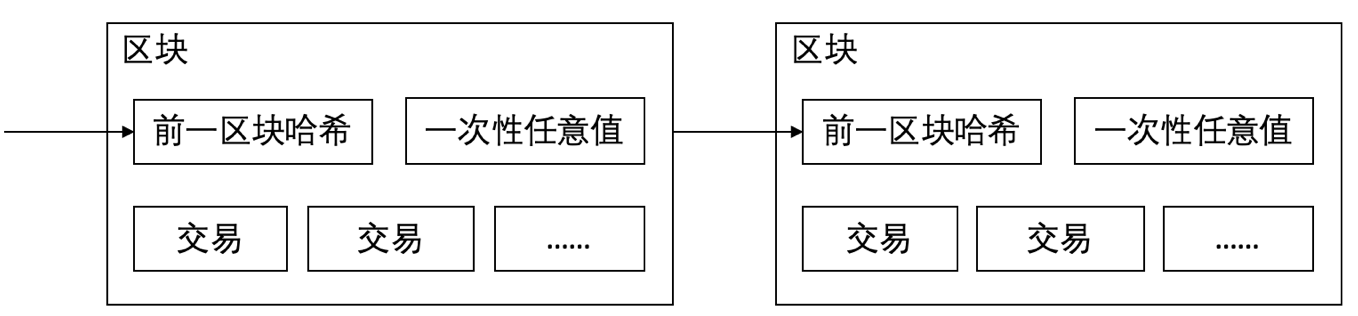5. 网络

1) 新交易被广播给全体节点。
2) 各个节点把新交易收集到区块内。
3) 各个节点进行工作，为它的区块寻找一个困难的工作量证明。
4) 当一个节点找到了一个工作量证明，它就把该区块广播给全体节点。
5) 节点接受该区块，仅当其中的所有交易都有效并且未被花费的情况下。
6) 节点通过在链上工作，使用已接受区块的哈希作为上一个哈希，创建下一个区块，以表示它们对该区块的接受。

6. 激励

7. 回收磁盘空间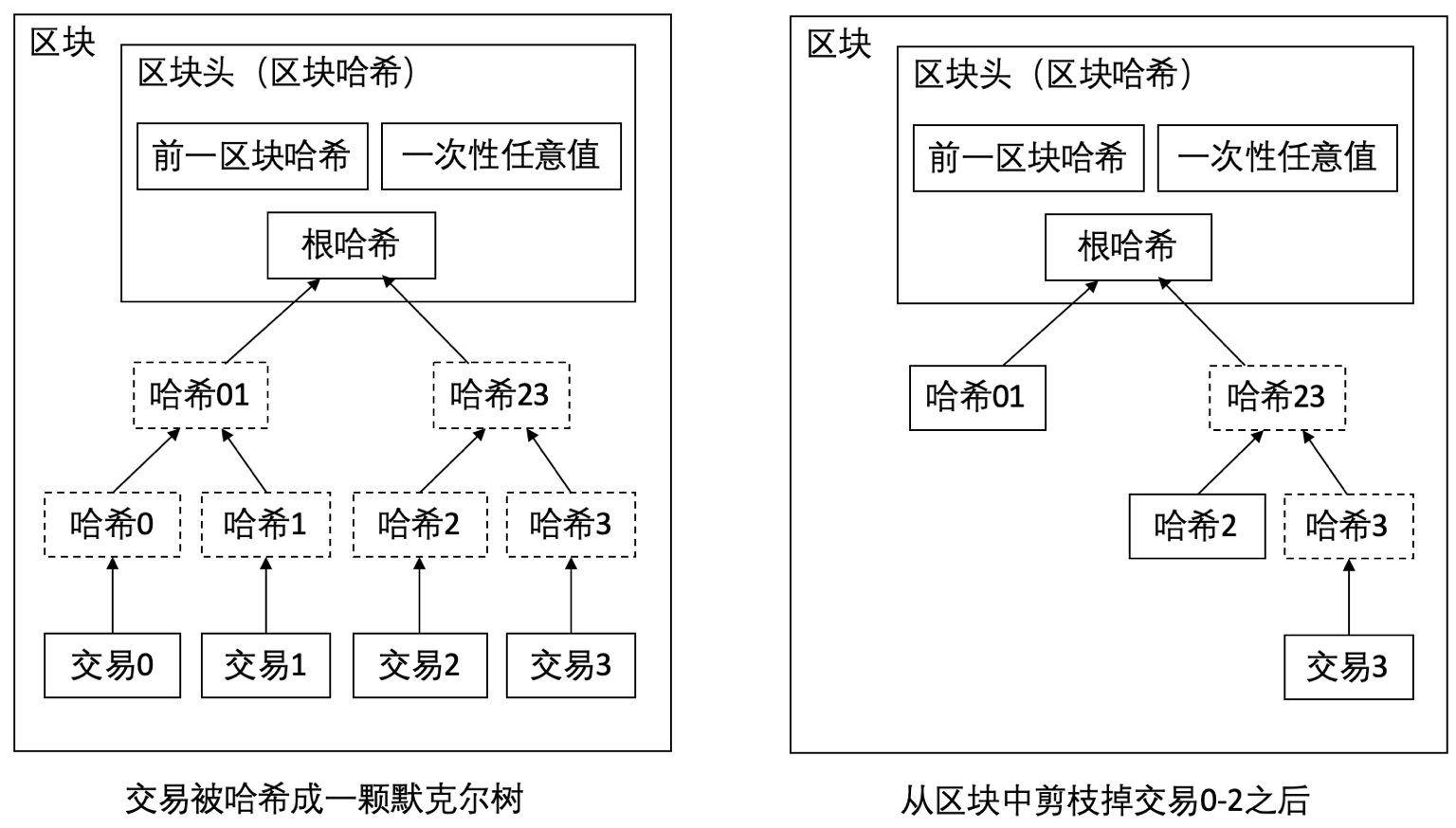8. 简化支付验证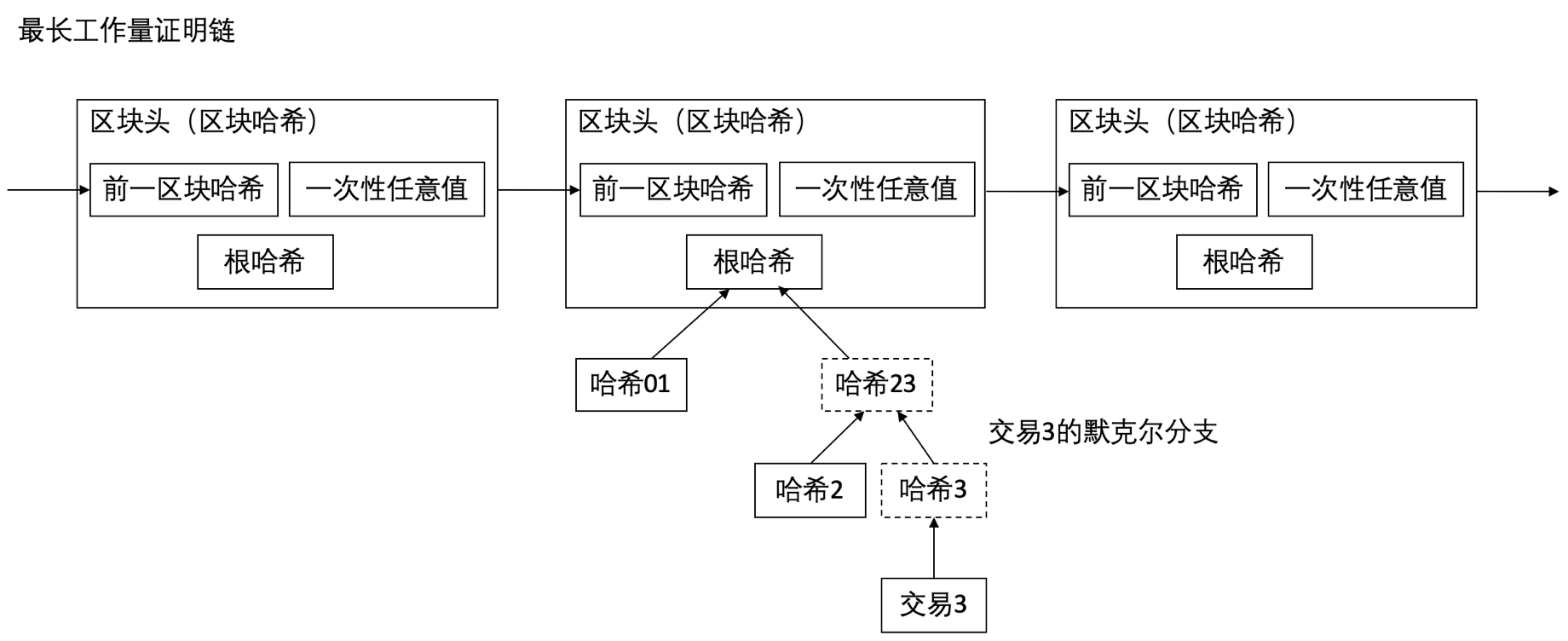9. 合并和分割价值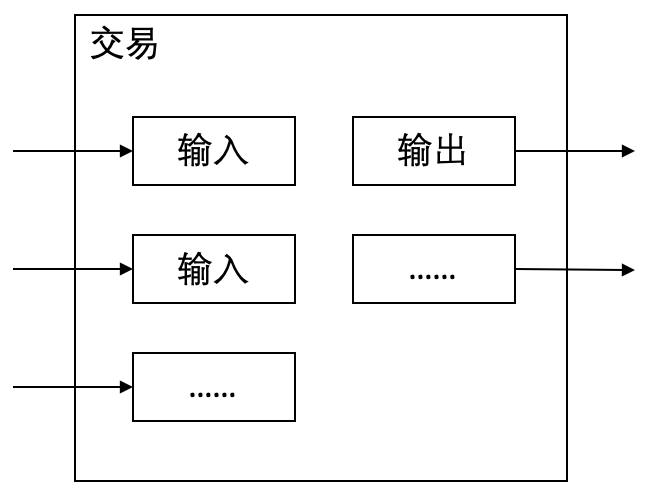10. 隐私11. 计算

p = 诚实节点发现下一区块的概率
q = 攻击者发现下一区块的概率
qz = 攻击者从落后z个区块处最终追上诚实链的概率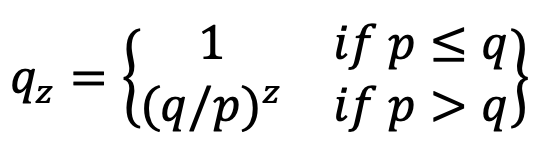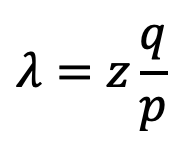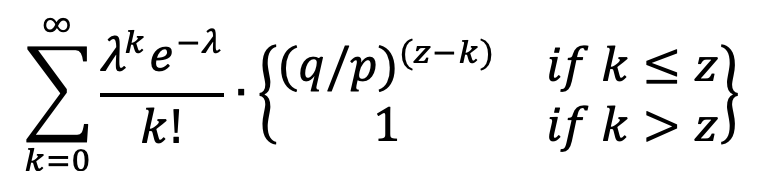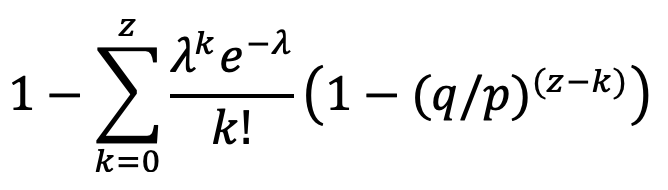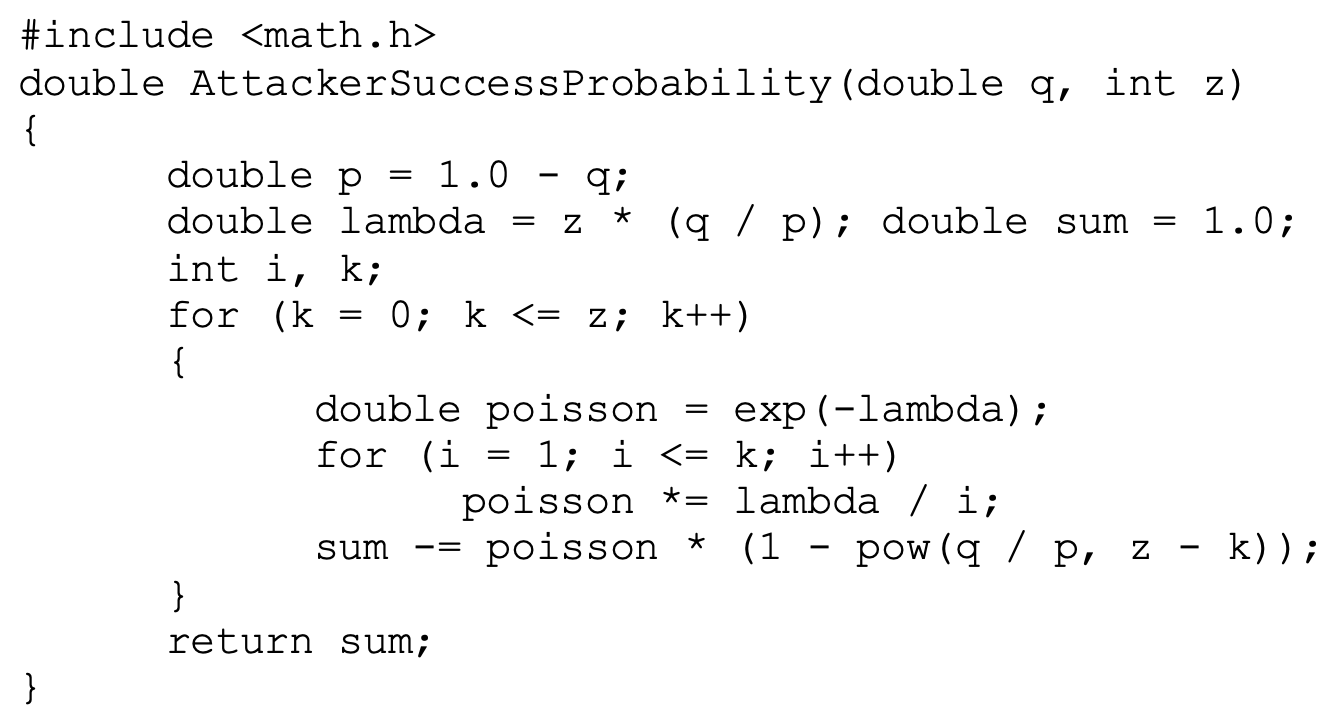q=0.1
z=0 P=1.0000000
z=1 P=0.2045873
z=2 P=0.0509779
z=3 P=0.0131722
z=4 P=0.0034552
z=5 P=0.0009137
z=6 P=0.0002428
z=7 P=0.0000647
z=8 P=0.0000173
z=9 P=0.0000046
z=10 P=0.0000012

q=0.3
z=0 P=1.0000000
z=5 P=0.1773523
z=10 P=0.0416605
z=15 P=0.0101008
z=20 P=0.0024804
z=25 P=0.0006132
z=30 P=0.0001522
z=35 P=0.0000379
z=40 P=0.0000095
z=45 P=0.0000024
z=50 P=0.0000006

P < 0.001
q=0.10 z=5
q=0.15 z=8
q=0.20 z=11
q=0.25 z=15
q=0.30 z=24
q=0.35 z=41
q=0.40 z=89
q=0.45 z=340

12. 结论

 W. Dai, "b-money," http://www.weidai.com/bmoney.txt, 1998.
 H. Massias, X.S. Avila, and J.-J. Quisquater, " Design of a secure timestamping service with minimal trust requirements," In 20th Symposium on Information Theory in the Benelux, May 1999.
 S. Haber, W.S. Stornetta, " How to time-stamp a digital document," In Journal of Cryptology, vol 3, no 2, pages 99-111, 1991.
 D. Bayer, S. Haber, W.S. Stornetta, "Improving the efficiency and reliability of digital time-stamping," In Sequences II: Methods in Communication, Security and Computer Science, pages 329-334, 1993.
 S. Haber, W.S. Stornetta, "Secure names for bit-strings," In Proceedings of the 4th ACM Conference on Computer and Communications Security, pages 28-35, April 1997.
 A. Back, "Hashcash - a denial of service counter-measure,"
http://www.hashcash.org/papers/hashcash.pdf, 2002.
 R.C. Merkle, "Protocols for public key cryptosystems," In Proc. 1980 Symposium on Security and Privacy, IEEE Computer Society, pages 122-133, April 1980.
 W. Feller, "An introduction to probability theory and its applications," 1957.

*根据央行等部门发布的“关于进一步防范和处置虚拟货币交易炒作风险的通知”，本文内容仅用于信息分享，不对任何经营与投资行为进行推广与背书，请读者严格遵守所在地区法律法规，不参与任何非法金融行为。*